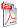Issue archive

https://doi.org/10.15255/KUI.2003.012
Published: Kem. Ind. 53 (10) (2004) 433—439
Paper reference number: KUI-12/2003
Paper type: Original scientific paper
Download paper:PDFDetermination of Mathematical Relation for Necessary Mass of Dough for Obtaining Bread with Defined Mass

M. Bocevska, T. Dimeski and I. Aldabas

##### Abstract

The bread was prepared from various types of flour: T-400, T-500, T-850 and T-1000 and manually divided into pieces of dough with exact mass of 650, 700, 750, 800, 900 and 1000 g. An increase of loss of mass with an increase of mass of dough, independently of the used type of flour was confirmed. However, the loss of mass decreased among the pieces of dough with the same mass, with an increase of type of flour. A strong linear correlation (R2 = 0.99) was confirmed between mass of dough and mass of bread after 3 hours of storage. Quantitative connection of mass of bread and necessary mass of dough was established as the equation of the first order, mDough = 45.654 + 1.0624 mCBread (3 hours storage). Its validity was tested and confirmed by following the data for mass of dough and bread of 600 g obtained of flour type T- 500 in industrial process.

##### Keywords

mass of dough, mass of bread, mathematical relation